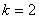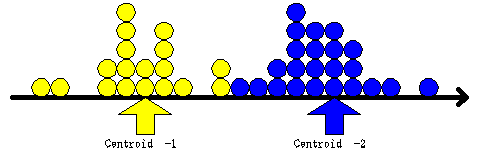## K Means Histogram

As you may guess, the minimum number of attribute is one. If the number of attribute is one, each example point represents a point in a distribution. The k-mean algorithm becomes the way to calculate the mean value of k distributions. Figure below is an example ofdistributions.If you set k=1, you will get the mean (arithmetic average) of your data.